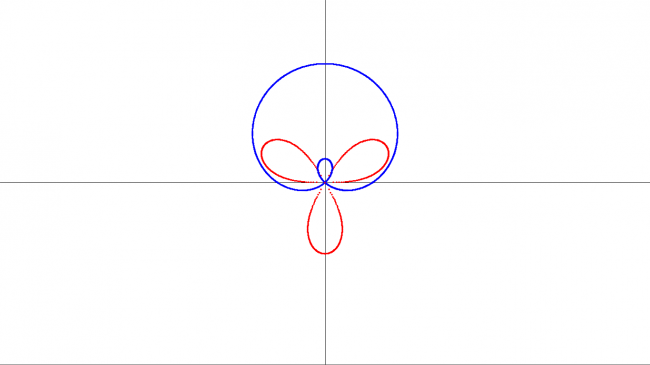#### Howdy, Stranger!

We are about to switch to a new forum software. Until then we have removed the registration on this forum.

# A program to plot polar equations

edited May 2017

Hello All !

I wrote this program to plot polar equations. For demonstration, I have plotted two equations. You can control various parameters like curve equations, line thickness, line color, background color, etc. Kindly give your feedback and suggestions. You can use it for your work, if you like...

Many Thanks!

``````float t=0,w,h;
float r=0,theta=0,r1=0;

void setup(){

fullScreen();
background(255);

h=height;
w=width;

/** DRAWING THE CO-ORDINATE AXES */
strokeWeight(1);
stroke(0);
line(0,h/2,w,h/2);
line(w/2,0,w/2,h);

/** LINE THICKNESS */
strokeWeight(3);
}

void draw(){

/** SHIFTING THE ORIGIN TO CENTER OF THE SCREEN */
translate(w/2,h/2);

/** ROTATING TO THE POLAR ANGLE */
rotate(theta);

/** EQUATIONS FOR THE TWO CURVES */
r=150*sqrt(sin(3*theta));
r1=100+150*(sin(theta));

/** PLOTTING THE FIRST CURVE */
stroke(255,0,0);
point(-r,0);

/** PLOTTING THE SECOND CURVE */
stroke(0,0,255);
point(-r1,0);

t+=0.25;
}
``````Tagged: